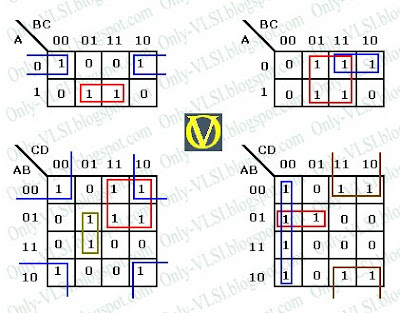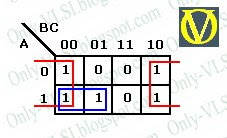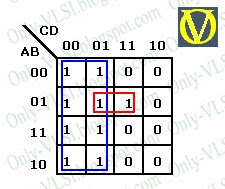### Boolean Expression Simplification

The k-map Method

The "Karnaugh Map Method", also known as k-map method, is popularly used to simplify Boolean expressions. The map method is first proposed by Veitch and then modified by Karnaugh, hence it is also known as "Veitch Diagram". The map is a diagram made up of squares (equal to 2 power number of inputs/variables). Each square represents a minterm, hence any Boolean expression can be represented graphically using a k-map.The above diagram shows two (I), three (II) and four (III) variable k-maps. The number of squares is equal 2 power number of variables. Two adjacent squares will differ only by one variable. The numbers inside the squares are shown for understanding purpose only. The number shown corresponds to a minterm in the the Boolean expression.

Simplification using k-map:
• Obtain the logic expression in canonical form.
• Identify all the minterms that produce an output of logic level 1 and place 1 in appropriate k-map cell/square. All others cells must contain a 0.
• Every square containing 1 must be considered at least once.
• A square containing 1 can be included in as many groups as desired.
• There can be isolated 1's, i.e. which cannot be included in any group.
• A group must be as large as possible. The number of squares in a group must be a power of 2 i.e. 2, 4, 8, ... so on.
• The map is considered to be folded or spherical, therefore squares at the end of a row or column are treated as adjacent squares.
The simplest Boolean expression contains minimum number of literals in any one in sum of products or products of sum. The simplest form obtained is not necessarily unique as grouping can be made in different ways.

Valid Groups

The following diagram illustrates the valid grouping k-map method.Simplification: Product of Sums

The above method gives a simplified expression in Sum of Products form. With slight modification to the above method, we can get the simplified expression in Product of Sums form. Group adjacent 0's instead of 1's, which gives us the complement of the function i.e. F'. The complement of obtained F' gives us the required expression F, which is done using the DeMorgan's theorem. See Example-2 below for better understanding.

Examples:

1. Simplify F(A, B, C) = Σ (0, 2, 4, 5, 6).

The three variable k-map of the given expression is:The grouping is also shown in the diagram. Hence we get,
F(A, B, C) = AB' + C'

2. Simplify F(A, B, C) = Σ (0, 2, 4, 5, 6) into Product of Sums.

The three variable k-map of the given expression is:The 0's are grouped to get the F'.
F' = A'C + BC

Complementing both sides and using DeMorgan's theorem we get F,
F = (A + C')(B' + C')

3. Simplify F(A, B, C, D) = Σ( 0, 1, 4, 5, 7, 8, 9, 12, 13)

The four variable k-map of the given expression is:The grouping is also shown in the diagram. Hence we get,
F(A, B, C, D) = C' + A'BD

### Setup and Hold TIme

Every flip-flop has restrictive time regions around the active clock edge in which input should not change. We call them restrictive because any change in the input in this regions the output may be the expected one (*see below). It may be derived from either the old input, the new input, or even in between the two. Here we define, two very important terms in the digital clocking. Setup and Hold time.
The setup time is the interval before the clock where the data must be held stable.The hold time is the interval after the clock where the data must be held stable. Hold time can be negative, which means the data can change slightly before the clock edge and still be properly captured. Most of the current day flip-flops has zero or negative hold time.

In the above figure, the shaded region is the restricted region. The shaded region is divided into two parts by the dashed line. The left hand side part of shaded region is the setup time period and the right hand side part is the hold time…

1. How do you convert a XOR gate into a buffer and a inverter (Use only one XOR gate for each)?

2. Implement an 2-input AND gate using a 2x1 mux.

3. What is a multiplexer?

A multiplexer is a combinational circuit which selects one of many input signals and directs to the only output.

4. What is a ring counter?

A ring counter is a type of counter composed of a circular shift register. The output of the last shift register is fed to the input of the first register. For example, in a 4-register counter, with initial register values of 1100, the repeating pattern is: 1100, 0110, 0011, 1001, 1100, so on.

5. Compare and Contrast Synchronous and Asynchronous reset.

Synchronous reset logic will synthesize to smaller flip-flops, particularly if the reset is gated with the logic generating the d-input. But in such a case, the combinational logic gate count grows, so the overall gate count savings may not be that significant. The clock works as a filter for small reset gl…

### Gate-Level Modeling

>> Introduction
>> Gate Primitives
>> Delays
>> Examples

Introduction

In Verilog HDL a module can be defined using various levels of abstraction. There are four levels of abstraction in verilog. They are:
Behavioral or algorithmic level: This is the highest level of abstraction. A module can be implemented in terms of the design algorithm. The designer no need to have any knowledge of hardware implementation.Data flow level: In this level the module is designed by specifying the data flow. Designer must how data flows between various registers of the design.Gate level: The module is implemented in terms of logic gates and interconnections between these gates. Designer should know the gate-level diagram of the design.Switch level: This is the lowest level of abstraction. The design is implemented using switches/transistors. Designer requires the knowledge of switch-level implementation details.
Gate-level modeling is virtually the lowest-level of abstraction, because t…